# Event-B Qualitative Probability User Guide

User:Son is in charge of the plug-in.

## Introduction

Event-B Qualitative Probability plug-in provides supports for reasoning about termination with probability 1 (almost-certain termination). For more details about the principles on Event-B Qualitative Probability, see the Qualitative Probability page.

## Installing and Updating

The plug-in is available through the main Rodin Update Site under Modelling Extension category.

## News

• 23.11.2011: Version 0.2.1 released for Rodin 2.3.*
• 10.04.2012: Version 0.2.1 is compatible with Rodin 2.4.*
• 14.05.2012: Version 0.2.1 is compatible with Rodin 2.5.*
• 09.03.2015: Version 0.2.2 is released with Rodin 3.x.x
• 09.10.2015: Version 0.2.3 is released
• 23.07.2017: Version 0.2.4 is released

## Technical References

• Thai Son Hoang. Reasoning about almost-certain convergence properties using Event-B. Science of Computer Programming, 81:108–121, February 2014 © Elsevier. SCP website (Please use this article as the reference for the plug-in).
• Extended version of the EASST paper (below).
• Probablistic convergence event with refinement
• Constraints on how (not-) to refine probabilistic events.
• Example: Duelling cowboys
• Example: Rabin's choice coordination.
• Example: Herman's probabilistic self-stabilization.
• E. Yilmaz, T.S. Hoang. Development of Rabin’s Choice Coordination Algorithm in Event-B. In Automated Verification of Critical Systems 2010, volume 35 of Electronic Communications of the EASST © EASST. EASST website
• Probablistic convergence event with refinement
• Constraints on how (not-) to refine probabilistic events.
• Example: Rabin's Choice Coordination.
• S. Hallerstede, T.S. Hoang. Qualitative Probabilistic Modelling in Event-B. In IFM 2007: Integrated Formal Methods, 6th International Conference Proceedings, Oxford, UK, July 2-5, 2007, volume 4591 of LNCS © Springer-Verlag. Springer website
• Initial idea about probabilistic convergence event.
• New modelling elements: Variant bound
• New proof obligations: PRV, BND, FINACT.
• Example: Resolve contention in IEEE 1395 (Firewire protocol).

## Usage

We illustrate the usage of the plug-in using the example of contention resolving (part of IEEE 1394 Firewire protocol). The description of the problem is as follows.

Two processes in contention use a probabilistic protocol to resolve the problem. In each step, each process probabilisitcally choose to communicate in either short or long delay. The contention is resolved when the processes choose different delays.

• Boolean variables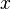$x$ and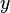$y$ represent the choice for each process: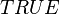$TRUE$ for short delay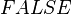$FALSE$ for long delay.
• Resolving contention is model as an event of the model with guard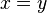$x = y$ (i.e. keep trying when the choices are identical).

### Probabilistic Modelling

• Set event resolve to be probabilistic convergence by setting its convergence attribute to be convergent and the probabilistic attribute of resolve from standard to probabilistic.
• Set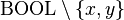$\Bool \setminus \{x, y\}$ as the variant of the model
• Create a new bound element (right-click on the machine name and choose Add Child/Event-B Bound Element).
• Set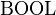$\Bool$ as the bound of the model
• Save the model

### Proof Obligations

• The model should have 3 proof obligations including resolve/PRV.
• The goal of proof obligation resolve/PRV is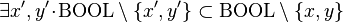$\exists x^\prime, y^\prime \qdot \Bool \setminus \{x^\prime, y^\prime\} \subset \Bool \setminus \{x, y\}$. With hypothesis$x = y$ (from the guard of the event), the proof obligation can be discharged by instantiating different values for$x^\prime$ and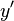$y^\prime$ (e.g.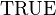$\True$ for$x^\prime$ and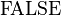$\False$ for$y^\prime$). Alternatively, the obligation can be interactively discharge using p1 (AterlierB Predicate Prover on lasso'd hypotheses) directly as shown below

## Additional features to be investigated/implemented

• Proof hints: Select event guards when creating proof obligations, such as PRV and BND
• Finer-grain for probabilisitc attribute. The probabilistic attribute might/should be attached to individual assignment and/or parameter of the event.